# Equivalence problem for systems of second-order ordinary differential equations

(diff) ← Older revision | Latest revision (diff) | Newer revision → (diff)

Let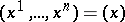,, andbe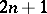coordinates in an open connected subsetof the Euclidean-dimensional space. Suppose that there is given a second-order system(a1)

for which each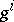isin a neighbourhood of initial conditions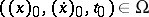.

Following D. Kosambi [a8], one wishes to solve the problem of finding the intrinsic geometric properties (i.e., the basic differential invariants) of (a1) under non-singular coordinate transformations of the type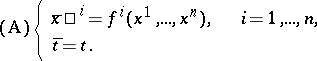A similar problem was solved by E. Cartan and S.S. Chern [a4], [a5], but in the real-analytic case with transformationsreplaced by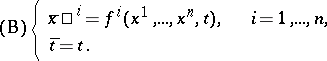Below, onlyis considered; see the references for.

Define the KCC-covariant differential of a contravariant vector fieldonby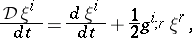(a2)

where the semi-colon indicates partial differentiation with respect to. Note that the Einstein summation convention (cf. Einstein rule) on repeated upper and lower indices is used throughout. Using (a2), equation (a1) can be re-expressed as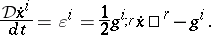(a3)

The quantityis a contravariant vector field onand constitutes the first KCC-invariant of (a1). It represents an "external force" .

If the trajectories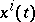of (a1) are varied into nearby ones according to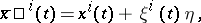(a4)

wheredenotes a constant with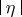small and theare the components of some contravariant vector defined along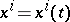, substitution of (a4) into (a1) and taking the limit as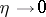results in the variational equations(a5)

where the comma indicates partial differentiation with respect to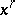. Using the KCC-covariant differential (a2), this can be re-expressed as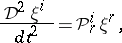(a6)

where(a7)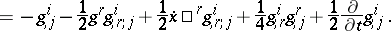The tensor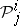is the second KCC-invariant of (a1). The third, fourth and fifth invariants are: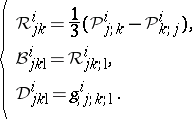(a8)

The main result of KCC-theory is the following assertion: Two systems of the form (a1) onare equivalent relative to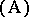if and only if the five KCC-invariant tensors,,,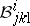, and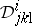are equivalent. In particular, there exist coordinates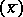for which the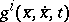all vanish if and only if all KCC-invariants are zero.

### Remarks.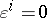if and only if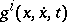are positively homogeneous of degree two in the variable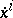. In this case, the structure ofmust accommodate possible non-differentiability in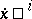. This happens in Finsler geometry, but not in affine and Riemannian geometries, where (a1) are geodesics or autoparallels of a linear connection whose coefficients are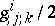. The latter are known as the coefficients of the Berwald connection in Finsler geometry, and of the Levi-Civita connection for Riemannian theory [a3], [a9], [a10]. Furthermore, in the Finsler case,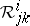,are the Berwald torsion and curvature tensors. Also,is the Douglas tensor, whose vanishing is necessary and sufficient for allto be quadratic in the variables. The latter is always zero in Riemannian and affine geometries, and also for Berwald spaces in Finsler theory [a6], [a3].

Finally, the KCC-invariants can be readily computed in each of the two following cases:

1)where,are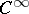functions ofonly. In this caseand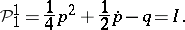The trajectories of this equation are Lyapunov stable if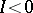, and unstable if;

2)where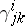are the coefficients of the Levi-Civita connection of a two-dimensional Riemannian metric,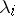are fixed constants and where the bracket on the right-hand side indicates no summation, [a3]. The KCC-invariants in the case whereare close to Riemannian, but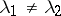has a significant effect on Lyapunov stability.

Further applications of KCC-theory can be found in [a2], [a1]. The equivalence problem can be found in a more general context in [a7], [a11].

How to Cite This Entry:
Equivalence problem for systems of second-order ordinary differential equations. Encyclopedia of Mathematics. URL: http://encyclopediaofmath.org/index.php?title=Equivalence_problem_for_systems_of_second-order_ordinary_differential_equations&oldid=16836
This article was adapted from an original article by P.L. Antonelli (originator), which appeared in Encyclopedia of Mathematics - ISBN 1402006098. See original article